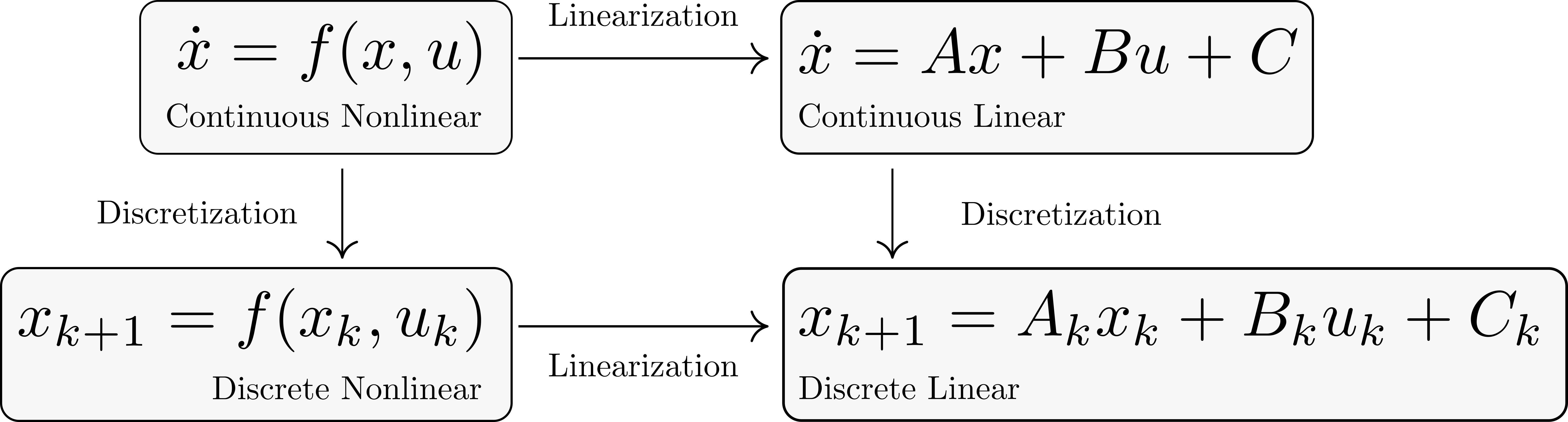# Do Discretization and Linearization Commute?

## Yes, up to first order (at least)

Posted on June 16, 2022

When taking a nonlinear, continuous time model and going to a linear, discrete time one (for example, when doing MPC), there are often questions about whether to linearize and then discretize, or discretize and then linearize, and up to what order. In other words, it is a question of whether or not the following diagram commutes:Start by considering a nonlinear continuous time model as: $\dot x = f(x,u),$ which we would like to convert to a linear discrete time model at some period $dt$.

Let’s start with the standard approach of linearizing first: $\dot x = f(\bar x, \bar u) + \frac{df}{dx}\Big\vert_{\bar x, \bar u}(x-\bar x) + \frac{df}{du}\Big\vert_{\bar x, \bar u}(u - \bar u).$ Now discretizing, we have: $x_{k+1} = x_k + \Bigg(f(\bar x, \bar u) + \frac{df}{dx}\Big\vert_{\bar x, \bar u}(x_k-\bar x) + \frac{df}{du}\Big\vert_{\bar x, \bar u}(u_k - \bar u)\Bigg)dt,$

On the other hand, if we first take a one step Euler discretization first: $x_{k+1} = x_k + f(x_k,u_k)dt,$ and now it’s first order linearization: \begin{align} x_{k+1} &= \bar x + f(\bar x,\bar u)dt + \Bigg((x_k-\bar x) + \frac{df}{dx}\Big\vert_{\bar x, \bar u}(x_k -\bar x) + \frac{df}{du}\Big\vert_{\bar x, \bar u}(u_k - \bar u)\Bigg)dt \notag \\ &= x_k + \Bigg(f(\bar x, \bar u) + \frac{df}{dx}\Big\vert_{\bar x, \bar u}(x_k-\bar x) + \frac{df}{du}\Big\vert_{\bar x, \bar u}(u_k - \bar u)\Bigg)dt, \notag \end{align} which agrees with what we had before.

In practice though, if you linearize and then discretize then you will likely use the exact discretezation (the discrete update map for LTI systems can be written down explicitly), and therefore may introduce less approximation error than first discretizing and then linearizing.